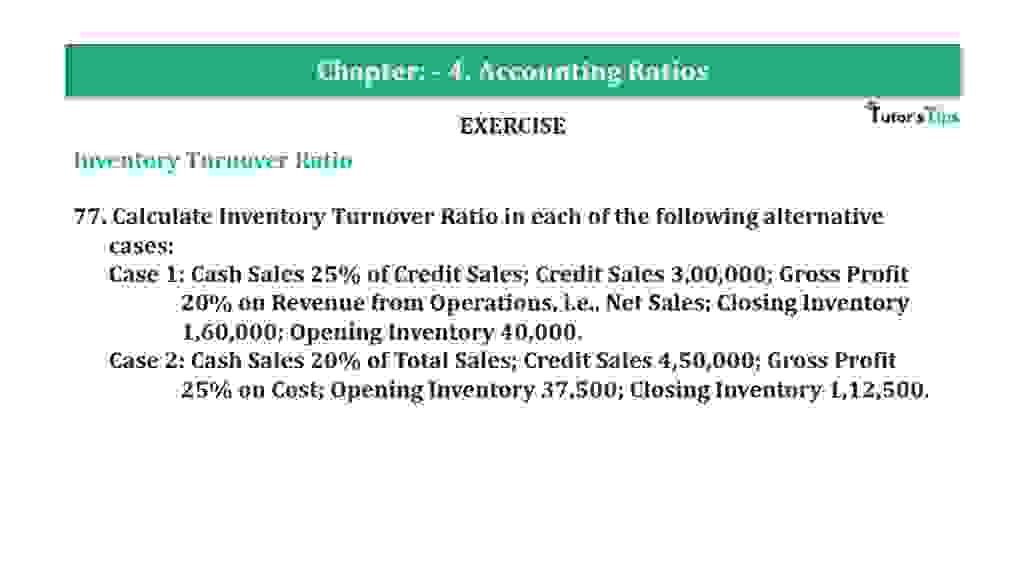# Question 77 Chapter 4 of +2-B – T.S. Grewal 12 ClassQuestion 77 Chapter 4 of +2-B

Interest Coverage Ratio

77. Calculate Inventory Turnover Ratio in each of the following alternative
cases:
Case 1: Cash Sales 25% of Credit Sales; Credit Sales 3,00,000; Gross Profit
20% on Revenue from Operations, i.e., Net Sales; Closing Inventory 1,60,000; Opening Inventory 40,000.
Case 2: Cash Sales 20% of Total Sales; Credit Sales 4,50,000; Gross Profit
25% on Cost; Opening Inventory 37,500; Closing Inventory 1,12,500.

### The solution of Question 77 Chapter 4 of +2-B: –

Case I:

 Credit Sales = Rs. 3,00,000 Cash Sales = @25% of Credit Sales = Rs. 3,00,000 x 25% Cash Sales = Rs. 75,000 Total Sales = Cash Sales + Credit Sales = Rs. 3,00,000 + Rs. 75,000 = Rs. 3,75,000 Gross Profit = Rs. 3,75,000 x 20% = Rs. 75,000 Cost of Goods Sold = Total Sales – Gross Profit = Rs. 3,75,000 – Rs. 75,000 = Rs. 3,00,000

 Inventory Turnover Ratio = Opening Inventory + Closing Inventory Average Inventory
 Inventory Turnover Ratio = Rs. 40,000 + Rs. 1,60,000 2 = Rs. 1,00,000
 Inventory Turnover Ratio = Cost of Goods Sold Average Inventory
 Inventory Turnover Ratio = Rs. 3,00,000 Rs. 1,00,000 = 3 Times

Case II:

 Total Sales = X Cash Sales = X x 20/100 Cash Sales = 20x/100 Total Sales = Cash Sales + Credit Sales

 X = 20x +Rs.4,50,000 100

 100x = 20x + Rs. 4,50,00,000 100x – 20x = Rs. 4,50,00,000

 X = Rs. 4,50,00,000 80 x = Rs. 5,62,500

Cost of Goods Sold = y

 Gross Profit = Y   X 25 100

 = 25y 100

 25y = Rs. 5,62,500 – y 100
 Y+ 25y = Rs. 5,62,500 100

 125y = Rs. 5,62,500 100
 Y = Rs. 5,62,00,000 125 Y = Rs. 4,50,000

Cost of Goods Sold = Rs. 4,50,000

 Average Inventory = Opening Inventory + Closing Inventory 2

 Average Inventory = Rs. 37,500 + Rs. 1,12,500 2 = Rs. 75,000

 Inventory Turnover Ratio = Cost of Goods Sold Average Inventory

 Inventory Turnover Ratio = Rs. 4,50,00 Rs. 75,000 = 6 Times

Balance Sheet: Meaning, Format & Examples

Thanks, Please Like and share with your friends

Comment if you have any question.

Also, Check out the solved question of previous Chapters: –

### T.S. Grewal’s Double Entry Book Keeping (Vol. II: Accounting for Companies)T.S. Grewal’s Analysis of Financial Statements